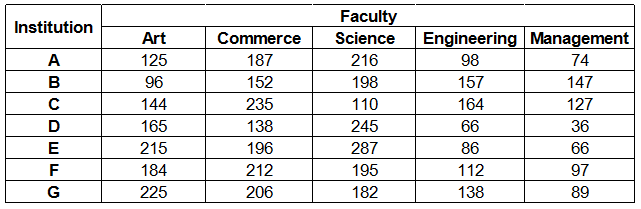### IBPS Clerk 2018 Question 17

Instructions

Study the following table carefully and answer the questions given below :
Number of students studying in different faculties in Seven institutionsQuestion 17

# Out of the total students of the institute D, approximately, what percentage of students are studying Management ?

Solution

Number of management students in institute D = 36

Total number of students in institute D

= 165 + 138 + 245 + 66 + 36 = 650

=> Required % = $$\frac{36}{650} \times 100$$

= $$\frac{72}{13} = 5.5 \%$$

$$\approx 5 \%$$